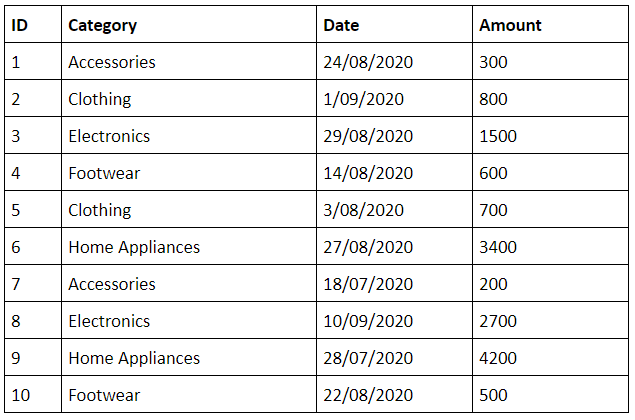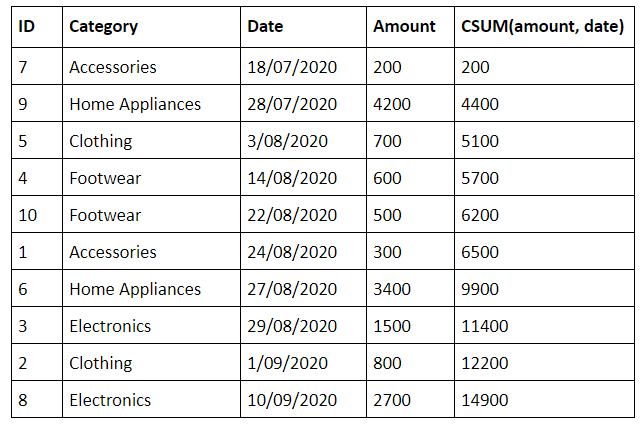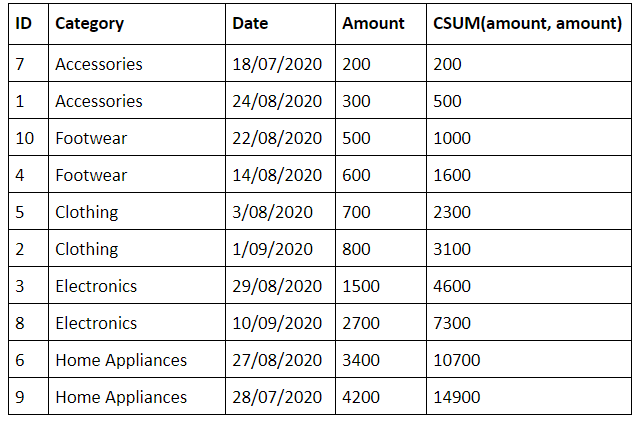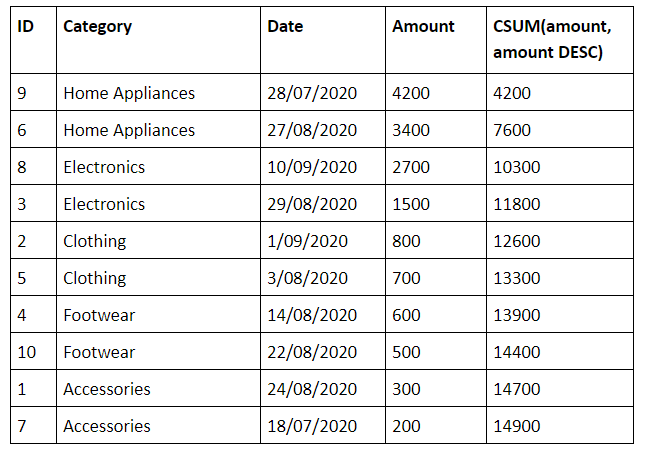OLAP ( Online Analytical Processing) functions helps in creating standard calculated measures like rank, aggregates, cumulative aggregates, etc. They take multiple elements from a list, apply a transformation on it, and returns a new list. These are mostly applied to numeric columns. They are highly essential for financial datasets or datasets that have a huge number of rows and columns. Oracle Teradata provides several OLAP functions to apply transformations on data. One of the important functions is the CSUM (Cumulative Sum). In this post, we will give a detailed explanation of what CSUM is and how it can be used.

## What is CSUM?

CSUM computes the cumulative sum of a value expression for each row over an ordered dataset. It performs a sum on the values of each row. It is also known as calculating the running sum.

Syntax

CSum(value_expression, sort_expression_list)

Value_expression is the scalar numeric column for which the running sum should be calculated.

Sort_expression_list is a list of column names separated by commas. It specifies the columns that have to be used to sort the values. Ascending (ASC) is the default sort direction.

The CSUM function sorts the dataset based on sort_expression_list first and then calculates the sum on the value_expression.

FREE Demo Class From Industry Experts - Enroll Now.!! Teradata Training

Example

Let’s take the below sales table and apply CSUM on it to find out the overall sale amount.To find the running sum of the over sales amount, execute the below query.

SELECT ID, category, date, amount, CSUM(amount, date) from sales;

The result of the query would be as follows.Don’t forget that we should give both the values for the CSUM function.

Sort and CSUM on the same column

We can also give the same column for ordering the table and applying CSUM. From the above example, let’s order by the amount column and apply CSUm on the same amount column. Here is the query for it.

• Lifetime LMS & Faculty Access
• Real-world & Project Based Learning

SELECT ID, category, date, amount, CSUM(amount, amount) from sales;

Below is the output for the query.You can observe that the table has been ordered by the amount column.

Sorting by descending order

As I told earlier, the default sorting order would be ascending order. We can change it to descending order through psssing DESC in the query. Here is how we can do it.

SELECT ID, category, date, amount, CSUM(amount, amount DESC) from sales;

Here is the output we will get when we execute the above query.You can observe that the above table has been ordered by the amount column in descending order.#### Conclusion

The CSUM function is more helpful than applying SUM on each row separately. For smaller tables, the difference might not be that much. But for larger tables, we can see a big difference. Now that you know how to use the CSUM function, try using it in your applications.

Weekday / Weekend Batches

Other Related articles:

#### Find our upcoming Teradata Training Online Classes

• Batch starts on 8th Feb 2023, Weekday batch

• Batch starts on 12th Feb 2023, Weekend batch

• Batch starts on 16th Feb 2023, Weekday batch